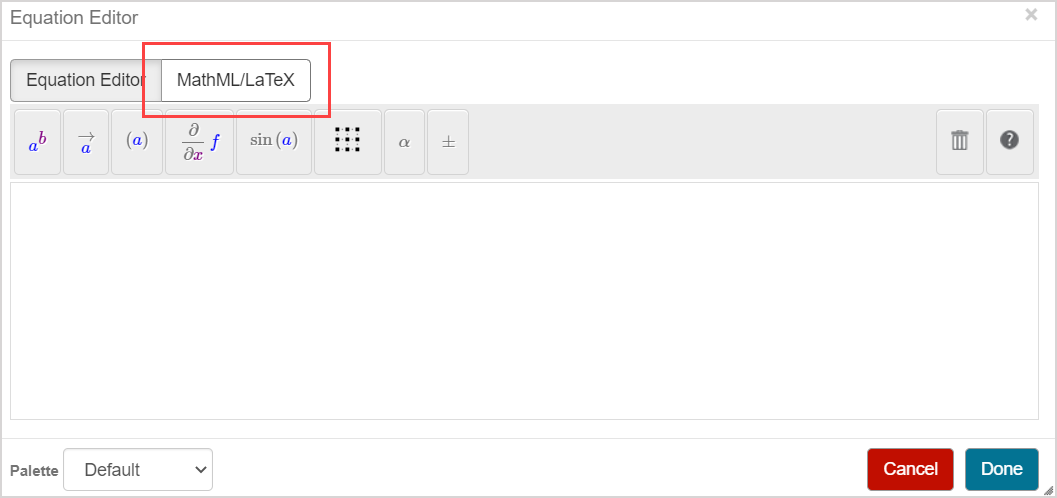# Work with LaTeX

You have the option to enter symbolic math expressions using MathML or LaTeX during question authoring.

Möbius implements LaTeX using the MathJax plugin which includes full support for macros in the AMSmath and AMSsymbols packages.

TIP: Check out MathJax support of LaTeX for a description of how Mathjax and LaTeX interact.

There are a few minor differences between the MathJax implementation by Möbius compared to your typical desktop setup:

• You must use the LaTeX math delimiters of $$...$$ for in-line math and $...$ for equations.
• The dollar sign (\$) is reserved for algorithmic variables in Möbius.
• Only Math-mode commands are supported (text formatting outside of Math-mode should be done by HTML code or the available tools in the Question Editor).
• You must ensure there are spaces around the less-than sign (<) and the greater-than sign (>), or use the custom commands of \lt and \gt instead of the symbols.

Keep these differences in mind during question authoring to enter your LaTeX code directly into the Question Text field of the Question Editor.

Alternatively, you can use the MathML/LaTeX tab within the Equation Editor to enter your LaTeX code.TIP: Check out the Enter MathML or LaTeX section of the Work with the Equation Editor (instructor) help topic for instructions on how to enter and preview your MathML or LaTeX code as scientific expressions and equations.

NOTE: The MathML/LaTeX tab of the Equation Editor is for LaTeX math-mode code only. Plain-text shouldn't be entered here.